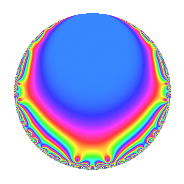# Properties

 Label 4010.2.a.gLevel 4010 Weight 2 Character orbit 4010.a Self dual yes Analytic conductor 32.020 Analytic rank 1 Dimension 2 CM no Inner twists 1

# Related objects

## Newspace parameters

 Level: $$N$$ = $$4010 = 2 \cdot 5 \cdot 401$$ Weight: $$k$$ = $$2$$ Character orbit: $$[\chi]$$ = 4010.a (trivial)

## Newform invariants

 Self dual: yes Analytic conductor: $$32.0200112105$$ Analytic rank: $$1$$ Dimension: $$2$$ Coefficient field: $$\Q(\sqrt{2})$$ Coefficient ring: $$\Z[a_1, a_2, a_3]$$ Coefficient ring index: $$1$$ Twist minimal: yes Fricke sign: $$1$$ Sato-Tate group: $\mathrm{SU}(2)$

## $q$-expansion

Coefficients of the $$q$$-expansion are expressed in terms of $$\beta = \sqrt{2}$$. We also show the integral $$q$$-expansion of the trace form.

 $$f(q)$$ $$=$$ $$q - q^{2} + \beta q^{3} + q^{4} + q^{5} -\beta q^{6} + 2 \beta q^{7} - q^{8} - q^{9} +O(q^{10})$$ $$q - q^{2} + \beta q^{3} + q^{4} + q^{5} -\beta q^{6} + 2 \beta q^{7} - q^{8} - q^{9} - q^{10} -2 q^{11} + \beta q^{12} + ( -2 - \beta ) q^{13} -2 \beta q^{14} + \beta q^{15} + q^{16} -\beta q^{17} + q^{18} -2 q^{19} + q^{20} + 4 q^{21} + 2 q^{22} + ( 2 - \beta ) q^{23} -\beta q^{24} + q^{25} + ( 2 + \beta ) q^{26} -4 \beta q^{27} + 2 \beta q^{28} -6 \beta q^{29} -\beta q^{30} -4 q^{31} - q^{32} -2 \beta q^{33} + \beta q^{34} + 2 \beta q^{35} - q^{36} + ( 6 - 3 \beta ) q^{37} + 2 q^{38} + ( -2 - 2 \beta ) q^{39} - q^{40} + 4 \beta q^{41} -4 q^{42} -8 \beta q^{43} -2 q^{44} - q^{45} + ( -2 + \beta ) q^{46} + ( -4 - 2 \beta ) q^{47} + \beta q^{48} + q^{49} - q^{50} -2 q^{51} + ( -2 - \beta ) q^{52} + ( 2 + 3 \beta ) q^{53} + 4 \beta q^{54} -2 q^{55} -2 \beta q^{56} -2 \beta q^{57} + 6 \beta q^{58} + ( 6 + 4 \beta ) q^{59} + \beta q^{60} + 8 q^{61} + 4 q^{62} -2 \beta q^{63} + q^{64} + ( -2 - \beta ) q^{65} + 2 \beta q^{66} + ( -4 - 5 \beta ) q^{67} -\beta q^{68} + ( -2 + 2 \beta ) q^{69} -2 \beta q^{70} + ( -8 + 2 \beta ) q^{71} + q^{72} + ( -2 - 6 \beta ) q^{73} + ( -6 + 3 \beta ) q^{74} + \beta q^{75} -2 q^{76} -4 \beta q^{77} + ( 2 + 2 \beta ) q^{78} + ( -12 + 4 \beta ) q^{79} + q^{80} -5 q^{81} -4 \beta q^{82} + ( -4 + 6 \beta ) q^{83} + 4 q^{84} -\beta q^{85} + 8 \beta q^{86} -12 q^{87} + 2 q^{88} -10 q^{89} + q^{90} + ( -4 - 4 \beta ) q^{91} + ( 2 - \beta ) q^{92} -4 \beta q^{93} + ( 4 + 2 \beta ) q^{94} -2 q^{95} -\beta q^{96} + ( -12 - \beta ) q^{97} - q^{98} + 2 q^{99} +O(q^{100})$$ $$\operatorname{Tr}(f)(q)$$ $$=$$ $$2q - 2q^{2} + 2q^{4} + 2q^{5} - 2q^{8} - 2q^{9} + O(q^{10})$$ $$2q - 2q^{2} + 2q^{4} + 2q^{5} - 2q^{8} - 2q^{9} - 2q^{10} - 4q^{11} - 4q^{13} + 2q^{16} + 2q^{18} - 4q^{19} + 2q^{20} + 8q^{21} + 4q^{22} + 4q^{23} + 2q^{25} + 4q^{26} - 8q^{31} - 2q^{32} - 2q^{36} + 12q^{37} + 4q^{38} - 4q^{39} - 2q^{40} - 8q^{42} - 4q^{44} - 2q^{45} - 4q^{46} - 8q^{47} + 2q^{49} - 2q^{50} - 4q^{51} - 4q^{52} + 4q^{53} - 4q^{55} + 12q^{59} + 16q^{61} + 8q^{62} + 2q^{64} - 4q^{65} - 8q^{67} - 4q^{69} - 16q^{71} + 2q^{72} - 4q^{73} - 12q^{74} - 4q^{76} + 4q^{78} - 24q^{79} + 2q^{80} - 10q^{81} - 8q^{83} + 8q^{84} - 24q^{87} + 4q^{88} - 20q^{89} + 2q^{90} - 8q^{91} + 4q^{92} + 8q^{94} - 4q^{95} - 24q^{97} - 2q^{98} + 4q^{99} + O(q^{100})$$

## Embeddings

For each embedding $$\iota_m$$ of the coefficient field, the values $$\iota_m(a_n)$$ are shown below.

For more information on an embedded modular form you can click on its label.

Label $$\iota_m(\nu)$$ $$a_{2}$$ $$a_{3}$$ $$a_{4}$$ $$a_{5}$$ $$a_{6}$$ $$a_{7}$$ $$a_{8}$$ $$a_{9}$$ $$a_{10}$$
1.1
 −1.41421 1.41421
−1.00000 −1.41421 1.00000 1.00000 1.41421 −2.82843 −1.00000 −1.00000 −1.00000
1.2 −1.00000 1.41421 1.00000 1.00000 −1.41421 2.82843 −1.00000 −1.00000 −1.00000
 $$n$$: e.g. 2-40 or 990-1000 Significant digits: Format: Complex embeddings Normalized embeddings Satake parameters Satake angles

## Inner twists

This newform does not admit any (nontrivial) inner twists.

## Twists

By twisting character orbit
Char Parity Ord Mult Type Twist Min Dim
1.a even 1 1 trivial 4010.2.a.g 2

By twisted newform orbit
Twist Min Dim Char Parity Ord Mult Type
4010.2.a.g 2 1.a even 1 1 trivial

## Atkin-Lehner signs

$$p$$ Sign
$$2$$ $$1$$
$$5$$ $$-1$$
$$401$$ $$-1$$

## Hecke kernels

This newform subspace can be constructed as the intersection of the kernels of the following linear operators acting on $$S_{2}^{\mathrm{new}}(\Gamma_0(4010))$$:

 $$T_{3}^{2} - 2$$ $$T_{7}^{2} - 8$$ $$T_{11} + 2$$